# Python | Plot different graphs using plotly and cufflinks

plotly is a Python library which is used to design graphs, especially interactive graphs. It can plot various graphs and charts like histogram, barplot, boxplot, spreadplot and many more. It is mainly used in data analysis as well as financial analysis. plotly is an interactive visualization library.

cufflink connects plotly with pandas to create graphs and charts of dataframes directly. choropleth is used to describe geographical plotting of USA. choropleth is used in the plotting of world maps and many more.

Let’s plot different types of plots like boxplot, spreadplot etc. using plotly and cufflinks.

Command to install plotly:

`pip install plotly `

`pip install cufflink`

Code #1: Show dataframe

 `# import all necessary libraries ` `import` `pandas as pd ` `import` `numpy as np ` ` `  `%` `matplotlib inline ` `from` `plotly ``import` `__version__ ` `import` `cufflinks as cf ` ` `  `from` `plotly.offline ``import` `download_plotlyjs, init_notebook_mode, plot, iplot ` ` `  `# to get the connection ` `init_notebook_mode(connected ``=` `True``) ` ` `  `# plotly also serves online, ` `# but we are using just a sample ` `cf.go_offline ` ` `  `# creating dataframes ` `df ``=` `pd.DataFrame(np.random.randn(``100``, ``4``), columns ``=``'A B C D'``.split()) ` ` `  `df2 ``=` `pd.DataFrame({``'Category'``:[``'A'``, ``'B'``, ``'C'``], ``'Values'``:[``32``, ``43``, ``50``]}) ` `df2.head() `

Output: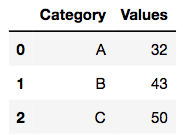Code #2: Normal Plot

 `# plotly function ` `df.iplot() `

Output: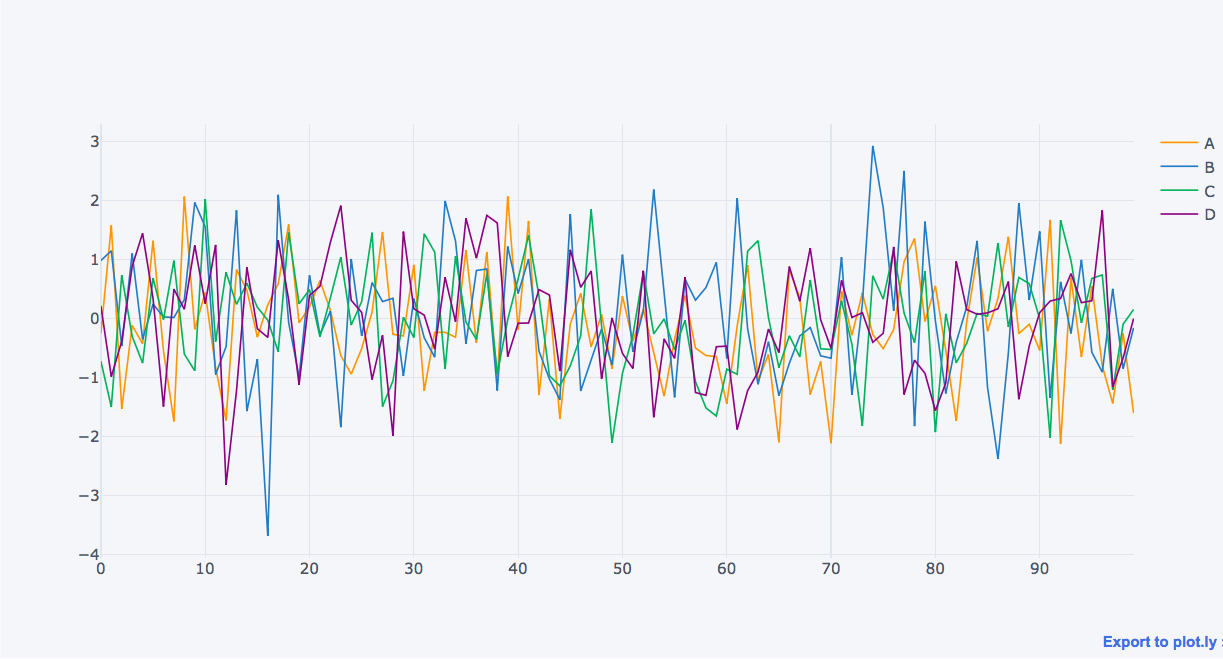Code #3: Scatter Plot

 `# markers are made to point in the graph ` `df.iplot(kind ``=``'scatter'``, x ``=``'A'``, y ``=``'B'``, mode ``=``'markers'``) `

Output: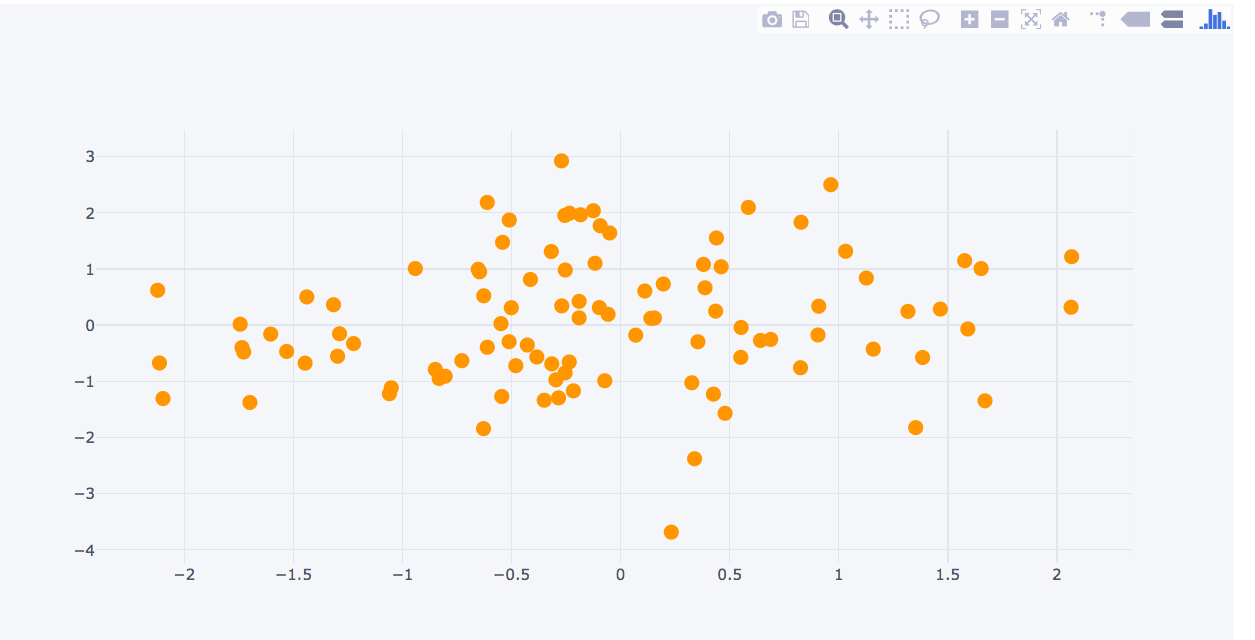Code #4: Box Plot

 `# boxplot ` `df.iplot(kind ``=``'box'``) `

Output: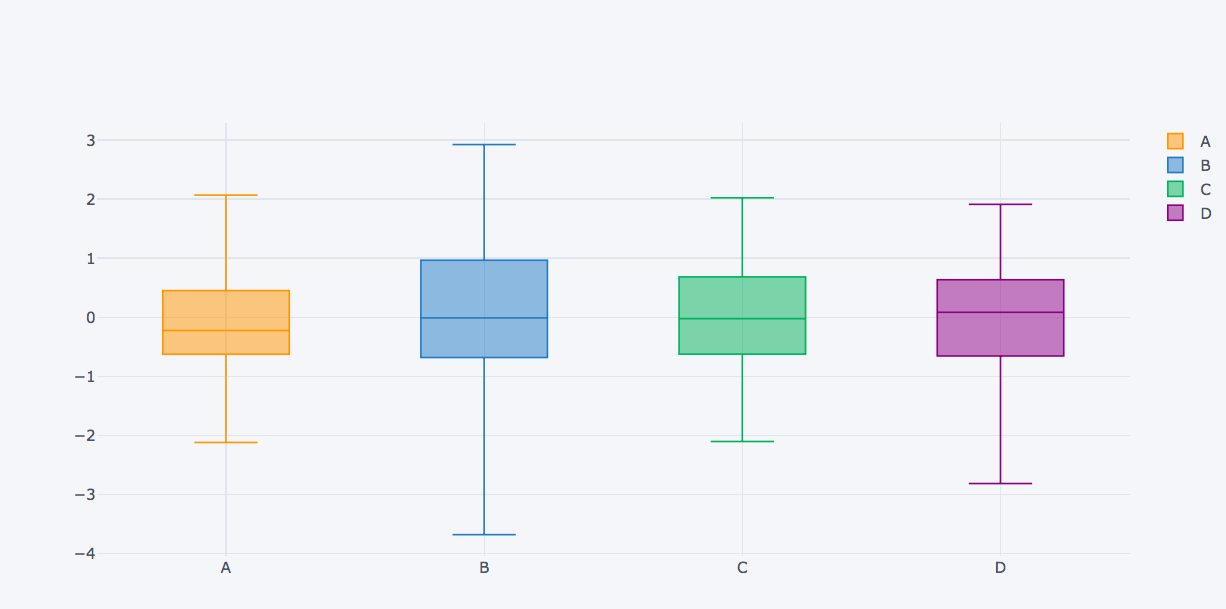Code #5: Plot dataframes

 `# creating dataframe with three axes ` `df3 ``=` `pd.DataFrame({``'x'``:[``1``, ``2``, ``3``, ``4``, ``5``], ` `                    ``'y'``:[``10``, ``20``, ``30``, ``20``, ``10``], ` `                    ``'z'``:[``5``, ``4``, ``3``, ``2``, ``1``]}) `

Output: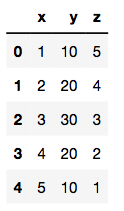Code #6: Surface plot

 `# surface plot ` `# colorscale:red(rd), yellow(yl), blue(bu) ` `df3.iplot(kind ``=``'surface'``, colorscale ``=``'rdylbu'``) `

Output: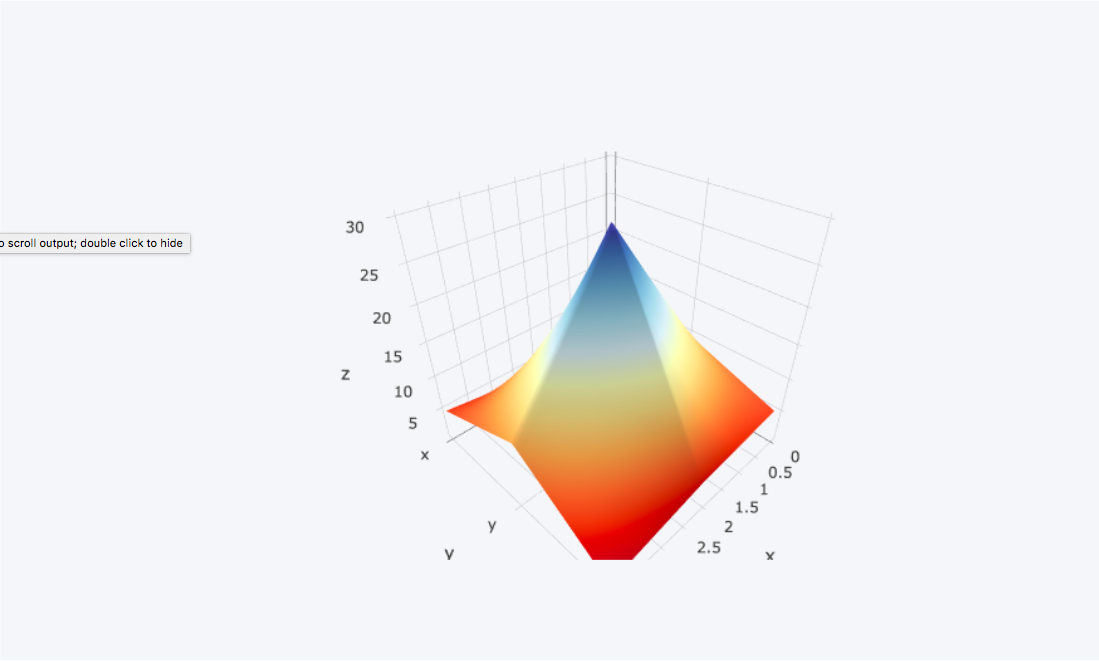My Personal Notes arrow_drop_upCheck out this Author's contributed articles.

If you like GeeksforGeeks and would like to contribute, you can also write an article using contribute.geeksforgeeks.org or mail your article to contribute@geeksforgeeks.org. See your article appearing on the GeeksforGeeks main page and help other Geeks.

Please Improve this article if you find anything incorrect by clicking on the "Improve Article" button below.

Article Tags :

2

Please write to us at contribute@geeksforgeeks.org to report any issue with the above content.